#### Electrical Conductivity and Real Semiconductors, Physics tutorial

Definition:

Electrical conductivity is basically the capability of a material to conduct the electrical current.

Electrical conductivity:

Electrical conductivity is, obviously, the quantity of primary interest in the semiconductors. Both electrons and holes contribute to electric current. Suppose first that a sample is strongly n-type and consists of only one kind of carrier: electrons. The conductivity can be treated according to the free- electron model:

σe = ne2τe/me

Here me is the effective mass and τe is the lifetime of the electron. To approximate the value for σe, we replace n = 1014 cm-3 that is eight orders in magnitude less than that in metals, and me = 0.l m. This leads to σe ~ 10-7 (µohm⋅cm)-1 that is a typical figure in semiconductors. However this is many orders of magnitude smaller than the value in a typical metal, here σe ~ 1(µohm⋅cm)-1, the conductivity in a semiconductor is still adequately large for the practical applications.

Semiconductor physicists frequently use the other transport coefficient: mobility. The mobility µe is stated as the proportionality coefficient between the electron drift velocity and the applied electric field, that is,

|Ve| = µeE,

Here, |Ve| is the absolute value of the velocity. Taking into account that je = - eneVe and je = σeE we find out that:

µe = eτe/me

As stated, the mobility is a measure of the rapidity of the motion of the electron in the field. The longer the life-time of the electron and the smaller its mass, the higher the mobility. We can now deduce electrical conductivity in terms of mobility. We can represent:

σe = neµe

Indicating that σe is proportional to µe. A typical value for µe might be obtained by replacing σe = 10-7 (µohm⋅cm)-1 and n = 1014 cm-3 in the above equation. This yield:

µe ~ 103 cm2 V-1 s-1

What we have stated regarding electrons in a strongly n-type substance can be carried over to the illustration of holes in a strongly p-type substance. The conductivity of the holes is represented by:

σh = pe2τh/mh = peµh

Here, µh is the hole mobility

Let us now treat the common case, in which both the electrons and holes are present. Whenever a field is applied, electrons drift opposite to the field and holes drift in the similar direction as the field. The currents of the two carriers are additive, though, and as a result the conductivities are also. Thus,

σ = σe + σh

That is, both the electrons and holes contribute to the currents. In terms of mobilities, one might write:

σ = neµe + peµh

The carrier's concentrations n and p might be different whenever the sample is doped, as illustrated before. And one or the other of the carriers might dominate, based on whether the semiconductor is n- or p-type. If the substance is in the intrinsic region, though, n = p, and the above equation becomes:

σ = ne (µe + µh)

Here, 'n' is the intrinsic concentration. Even now the two carriers don't contribute equally to the current. The carrier having the greater mobility generally the electron contributes the bigger share.

Dependence on temperature:

Conductivity mainly depends on temperature, and this dependence is often pronounced. Consider a semiconductor in the intrinsic region. Its conductivity is represented by the above equation. However in this condition the concentration 'n' rises exponentially with temperature. We might write the conductivity in the form:

σ = F (T) e(-Eg/2KT)

Here F(T) is a function that depends only weakly on the temperature. (This function based on the mobilities and effective masses of the carriers.) Therefore conductivity rises exponentially with temperature as illustrated in the figure shown below: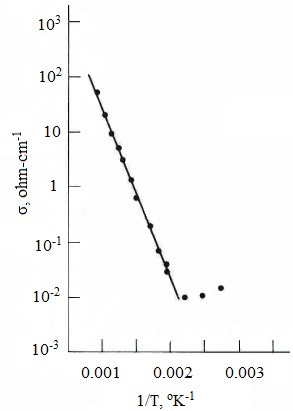This result can be employed to find out the energy gaps in semiconductors. In early days of semiconductors this was the standard method for determining the energy gap. These days, though, the gap is frequently computed by optical methods.

If the substance is not in the intrinsic region, its conductivity is represented by the general expression (σ = neµe + peµh). In that condition, the temperature dependence of the conductivity on 'T' is not generally as strong as indicated above. To recognize the reason for this, assume that the substance is extrinsic and strongly n-type. The conductivity is:

σ = neµe

However the electron concentration 'n' is now a constant equivalent to Nd, the donor (hole) concentration. And any temperature dependence present should be due to the mobility of the electrons or holes.

Mobility versus temperature:

Mobility of electrons or holes differs with temperature. In n-type semiconductor:

µe = eτe/me = ele/meve

Since the lifetime of the electron, or its collision time, varies with temperature, its mobility also varies with temperature. Normally, both lifetime and mobility diminish as the temperature rises.

The relaxation time is given by τe = le/ve, where le is the mean free path of the electron and ve is the drift velocity. The velocity of electrons is different based on their location in the conduction band. The electrons at the bottom of conduction band in a semiconductor obey the classical statistics and not the highly degenerate Fermi statistics prevailing in metals. The higher electrons are in the band, the greater their velocity.

We can assess the conductivity by supposing that ve is the average velocity. The average velocity can be estimated by employing the method of the kinetic theory of gases:

1/2 meve2 = 3/2 kT

This brings in a factor of T-1/2 dependence in the mobility:

µe = ele/me1/2 (3kT)1/2

The mean free path le as well based on the temperature, and in much similar way as it does in metals. le is found out by different collision methods acting on the electrons. Such methods are the collisions of electrons having thermally excited phonons and collisions with impurities. At high temperatures, at which collisions with phonons is the dominant factor, le is inversely proportional to temperature, that is, le ~ T-1. In that condition, mobility varies as µe ~ T-3/2. The figure below represents this for Ge.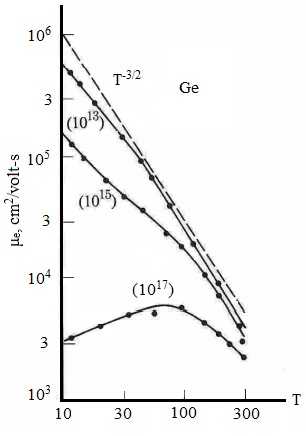The other significant scattering method in semiconductors is that of ionized impurities. If a substance is doped, the donors (or acceptors) lose their electrons (or holes) to the conduction band. The impurities are therefore ionized, and are quite effective in scattering the electrons (holes). At high temperatures this scattering is masked via the much stronger phonon method, however at low temperatures this latter method becomes weak and the ionized-impurity scattering steadily takes over.

Band structure of real semiconductors:

Till now, we have supposed the simplest possible band structure, namely, a conduction band of a standard form, centered at the origin, k = 0, and a valence band of a standard inverted form, as well centered at the origin. Such a simple structure is applicable for explaining most of the observed phenomena; however it doesn't represent the actual band structures of lots of common semiconductors. Only if one employs the actual band structure is it possible to get a quantitative agreement between experiments and theoretical analysis.

A material whose band structure comes close to the ideal structure is GaAs (figure shown below). The conduction band consists of a minimum at the origin k = 0, and the region close to the origin is well symbolized through a quadratic energy dependence, E(k) = (h/2π)2k2/me, here me = 0.072m. As the electrons are most probable to populate this region, one can symbolize this band through a single effective mass.

Note, though, that as 'k' increases, the energy E(k) is no longer quadratic in k, and such states might no longer by represented by a single, unique effective mass. In specific, that the next-higher energy minimum takes place all along the  direction. The dependence of energy on k in the neighborhood of this secondary minimum is quadratic, and therefore an efficient mass might be stated locally, however its value is much more than that of the primary minimum (at the center). The real value is 0.36m. Due to the cubic symmetry there are six equivalent secondary minima, or valleys, in all along the  directions.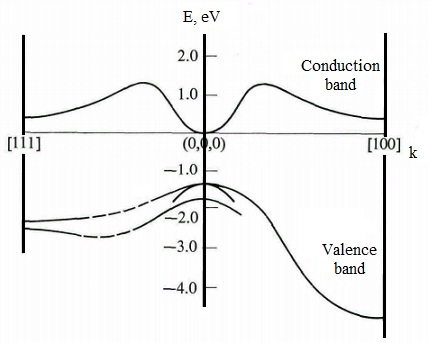Such secondary valleys don't play any role under most conditions, as the electrons generally engage only the central, or primary, valley. In such conditions, these secondary valleys might be disregarded altogether. There are as well other secondary valleys in the  directions, as shown in the figure above. These are higher than the  valleys, and therefore are even less likely to be populated via electrons.

The valence band is as well described in the figure shown above. Here this is composed of three closely spaced sub-bands. As the curvatures of the bands are different, so are the effective masses of the corresponding holes. One speaks of light holes and heavy holes.

Other III-V semiconductors have band structures quite identical to that of GaAs.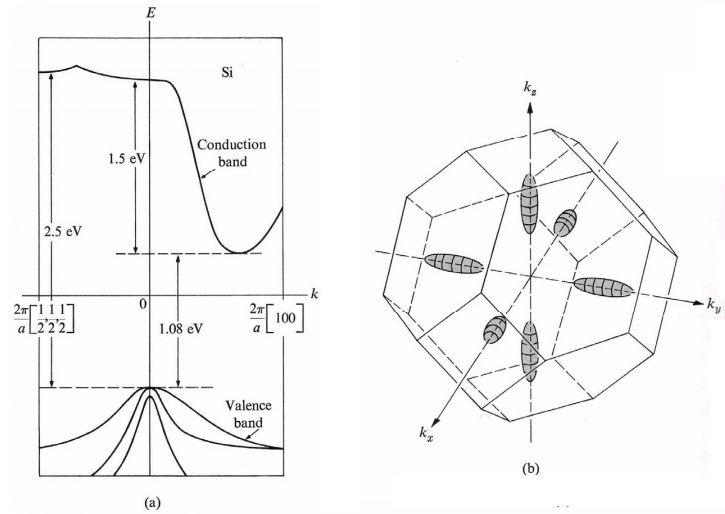Fig: (a) Band structure of Si plotted all along the  and  directions, (b) Ellipsoidal energy surfaces corresponding to primary valleys all along the  directions.

The first part of the figure above represents the band structure of Si. An interesting characteristic is that the conduction band consists of its lowest (primary) minimum not at k = 0. The minimum lies all along the  direction, at around 0.85 the distance from the center to the edge of the zone. It will be noted that the bottom of the conduction doesn't lie directly above the top of the valence band. This kind of semiconductors is termed as indirect gap semiconductors. These must be differentiated from direct gap semiconductors like GaAs. Due to the cubic symmetry, there are in reality six equivalent primary valleys located all along the  directions. These are described in the second part of the above figure. The energy surfaces at such valleys are composed of elongated ellipsoidal surfaces of revolution, whose axes of symmetry are all along the  directions. There are two different effective masses that correspond to these surfaces: the longitudinal and the transverse effective masses. The longitudinal mass is ml = 0.97m, whereas the two similar transverse masses are mt = 0.19m. The mass anisotropy ratio is around 5.

The valence band in silicon is symbolized by three different holes (first part of the above figure). One of the holes is heavy (mh = 0.5m), and the other two are light. The energy gap in Si, from the top of the valence band to the bottom of the conduction band, is equivalent to 1.08 eV. The fact that the bottom of the conduction doesn't lie directly over the top of the valence band, is irrelevant to the statement of the band gap.

Excitons:

An electron and a hole might be bound altogether via their attractive coulomb interaction, just as an electron is bound to a part to form a neutral hydrogen atom. The bound electron-hole pair is termed as an exciton, figure shown below. An exciton can move although the crystal and transport energy; it doesn't transport charge as it is electrically neutral. This is similar to positronium that is made up of from an electron and a positron.

Excitons can be made in each and every insulating crystal. All Excitons are unstable with respect to the ultimate recombination method in which the electron drops into the hole.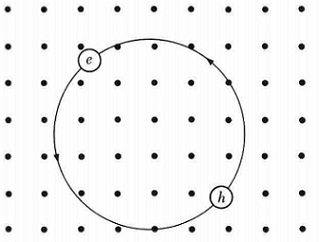The binding energy of the exciton can be evaluated though optical transitions from the valence band, by the difference between the energy needed to make an exciton and the energy to create a free electron and free hole,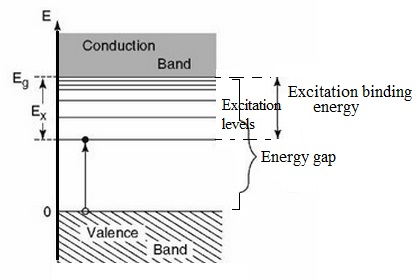Energy levels of the exciton can be computed as follows. Consider an electron in the conduction band and a hole in the valence band. The electron and hole attract one other through the Coulomb potential

V(r) = - e2r,

Here, 'r' is the distance between the particles and 'ε' is the suitable dielectric constant. There will be bound states of the Excitons system having total energies lower than the bottom of the conduction band. The problem is the hydrogen atom problem if the energy surfaces for the electron and hole are spherical and nondegenerate. The energy levels are represented by:

En = EC - [e4μ/2ε2(h/2π)2n2]

Here 'n' is the principal quantum number and 'μ' is the reduced mass:

1/μ = 1/me + 1/mh

Made up of from the effective masses of the electron and hole. The Excitons ground state energy is obtained on setting n = 1 in equation En = EC - [e4μ/2ε2(h/2π)2n2]; this is the ionization energy of  the Excitons.

Tutorsglobe: A way to secure high grade in your curriculum (Online Tutoring)